# 1123. Data Structure - N-ary TreeN-ary and Nary

Implement and traverse N-ary Tree.

## 1. Binary Search Tree

### 1.1 Definition of BST

Binary Search Tree(BST) is a node-based binary tree data structure which has the following properties:

• The left subtree of a node contains only nodes with values lesser than the node’s value.
• The right subtree of a node contains only nodes with values greater than the node’s values.
• The left and right subtree each must also be a binary search tree.
• There must be no duplicate nodes.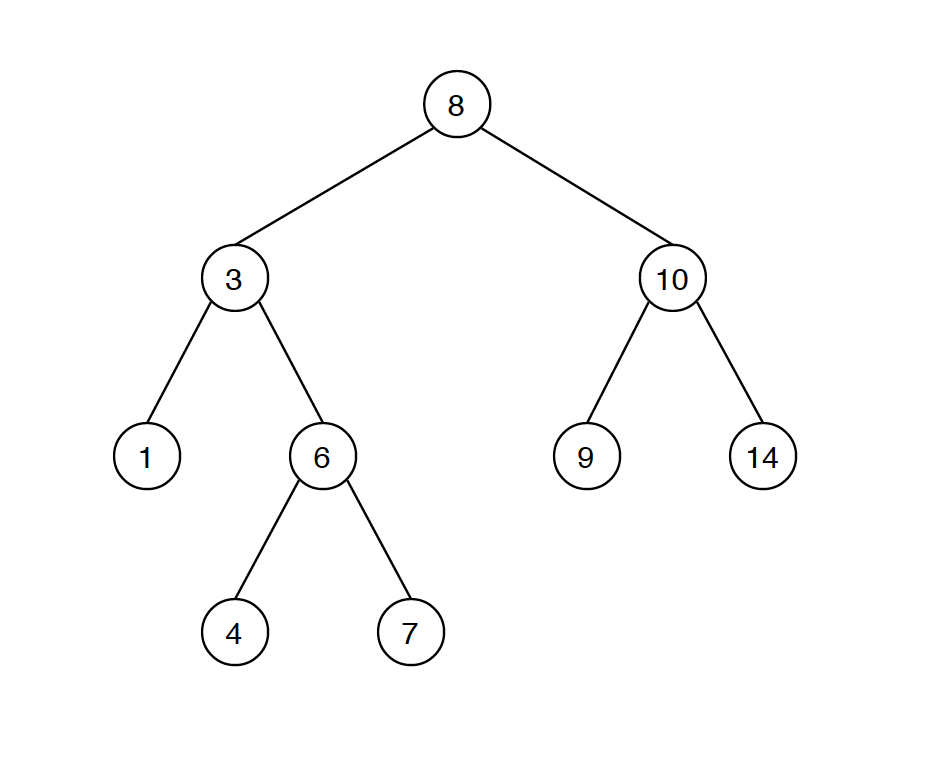### 1.2 Common Operations on BST

• Search - $O(\log{}n)$
• Insertion - $O(\log{}n)$
• Deletion - $O(\log{}n)$

Given a binary search tree as follows, search node with value 7.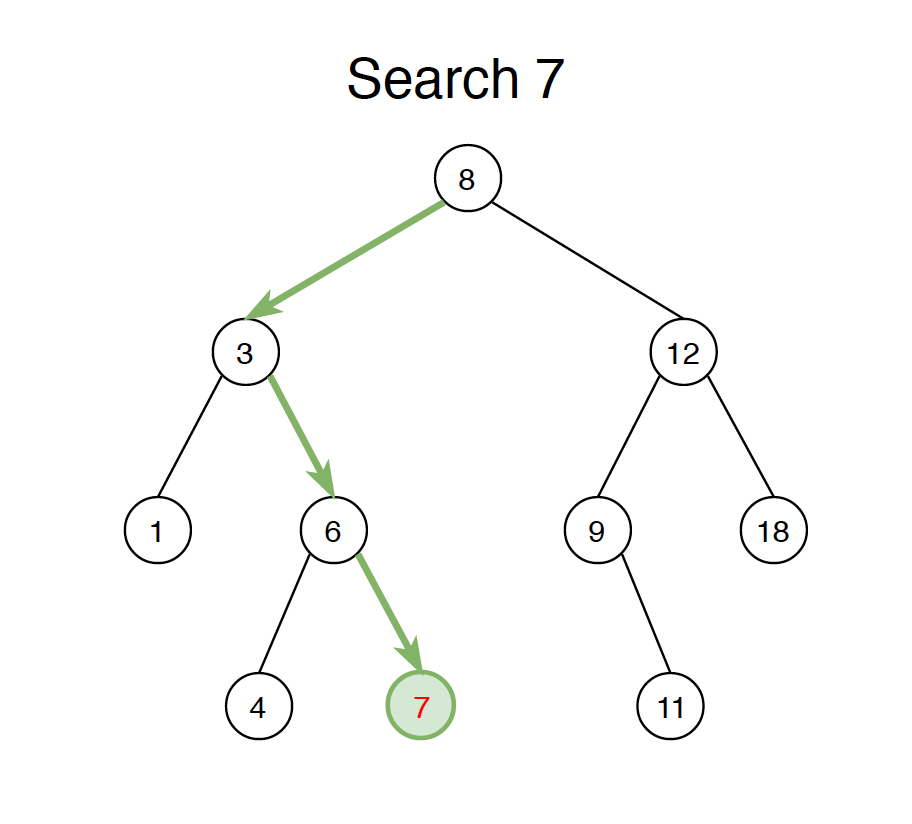Binary Search Tree is constructed with nodes recursively. The following example shows how the node is defined.

public class BSTNode {
public int val;
public BSTNode left, right;

public BSTNode(int value) {
this.val = value;
}
}


To search a given value in Binary Search Tree, we first compare it with root, if the key is present at root, we return root. If the value is greater than root’s value, we search further in the right subtree. Otherwise we search further in the left subtree.

public boolean search(int val) {
BSTNode current = root;
while ( current != null) {
if (current.val == val) {
return true;
} else if (current.val > val) {
current = current.left;
} else {
current = current.right;
}
}
return false;
}


## 3. Insertion

Given a binary search tree as follows, insert new value 5 into this tree.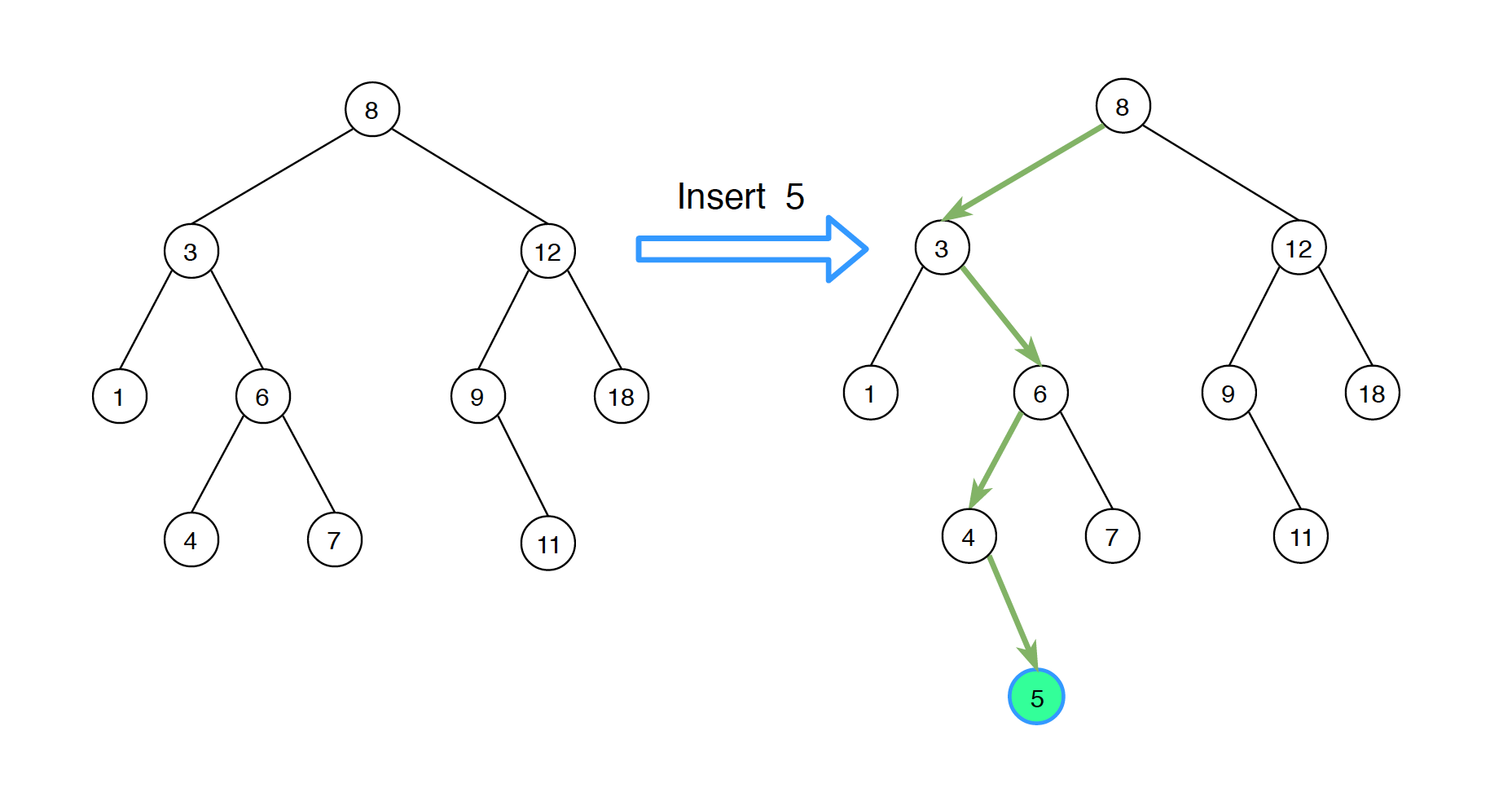A new value is always inserted at leaf. Similar with the search operation, we start searching the given value from root till we hit a leaf node. Once a leaf node is found, the new node is added as a child of the leaf node. At each step, we compare the given value with the node value to determine whether to go left or right.

public void insert(int val) {
BSTNode newNode = new BSTNode(val);
if (root == null) {
root = newNode;
return;
}
BSTNode current = root;
while (true) {
if (val < current.val) {
if (current.left == null) {
current.left = newNode;
return;
}
current = current.left;
} else {
if (current.right == null) {
current.right = newNode;
return;
}
current = current.right;
}
}
}


## 4. Deletion

There are three cases when deleting a node from Binary Search Tree.

• Node is leaf, has no children.
• Node has only one child.
• Node has two children.

### 4.1 Node Has No Children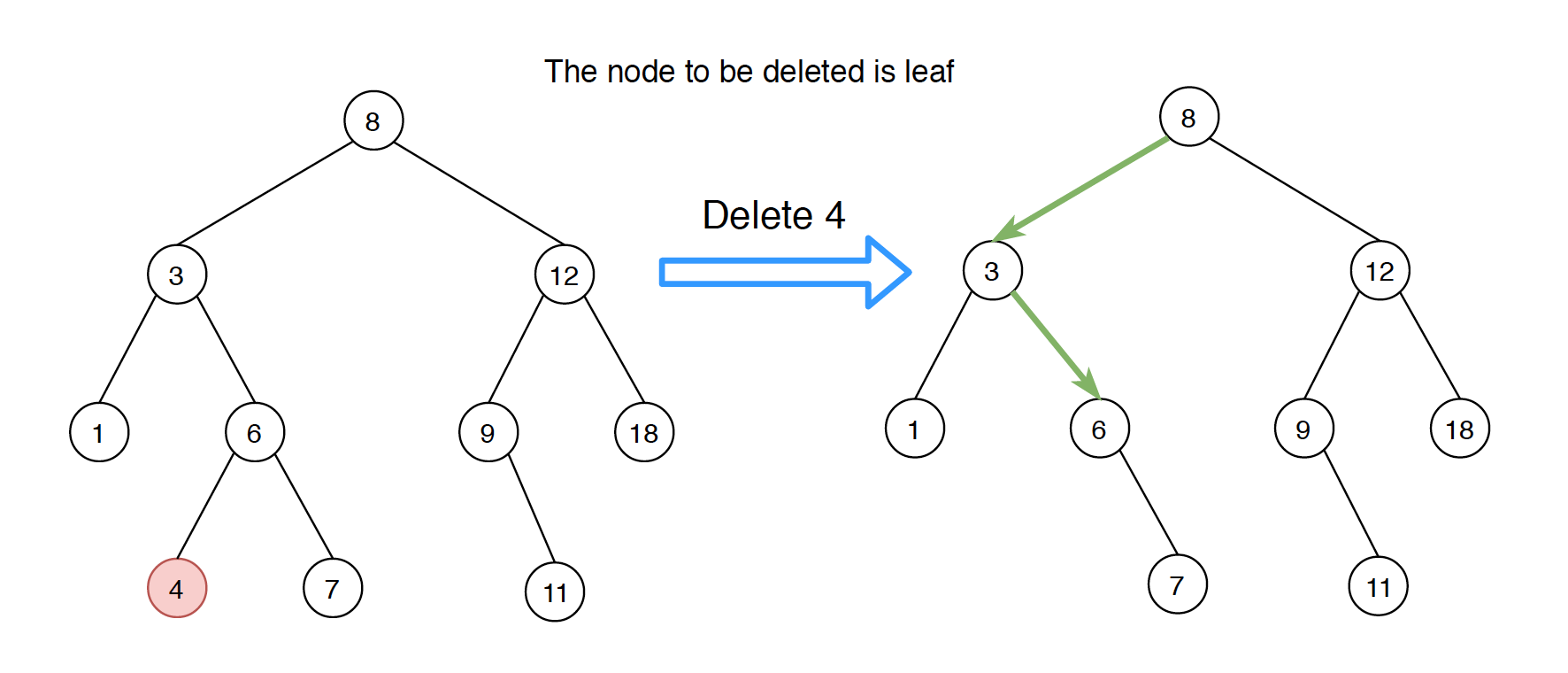The solution is easy, simply remove the node from the tree.

### 4.2 Node Has One Child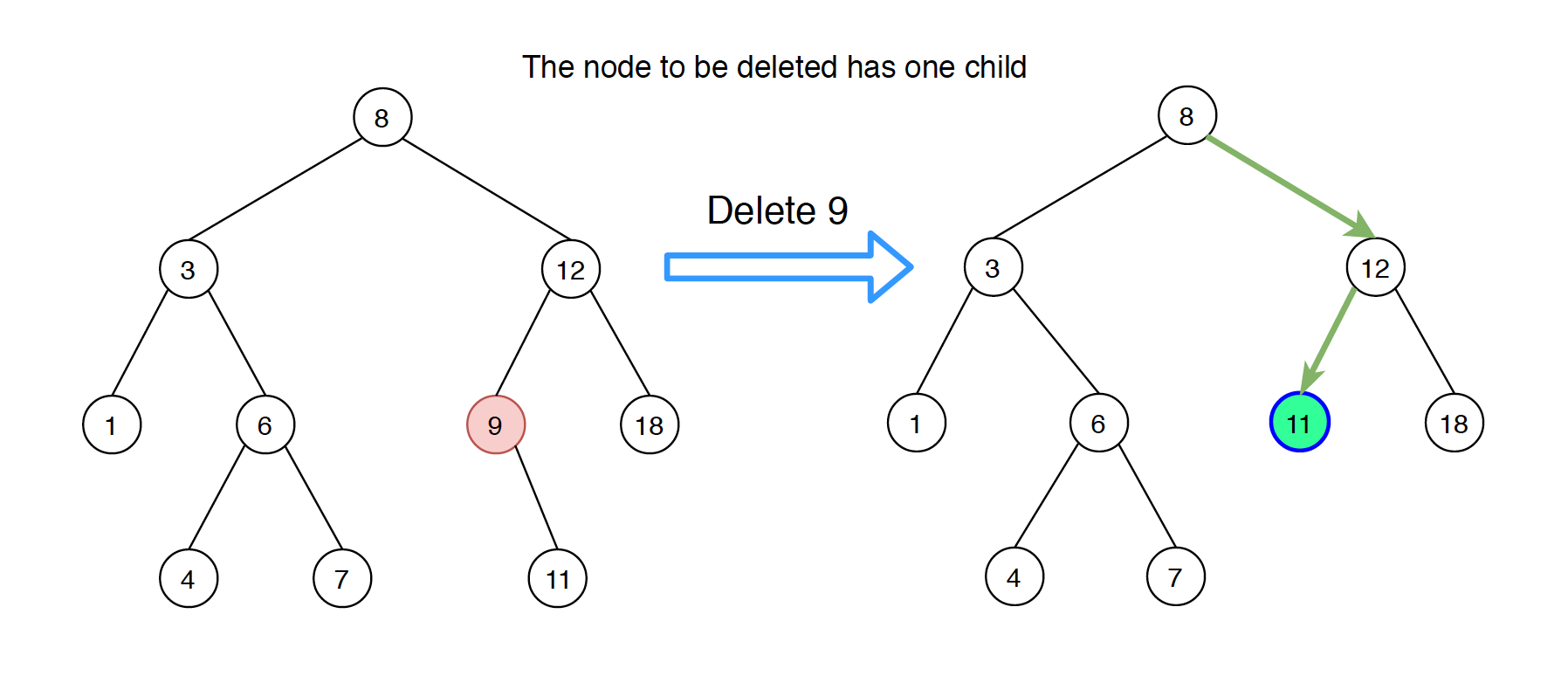If node has only one child, then replace this node with its child.

### 4.3 Node Has Two Children

This case is more complex, and we have two options.
1) Populate successor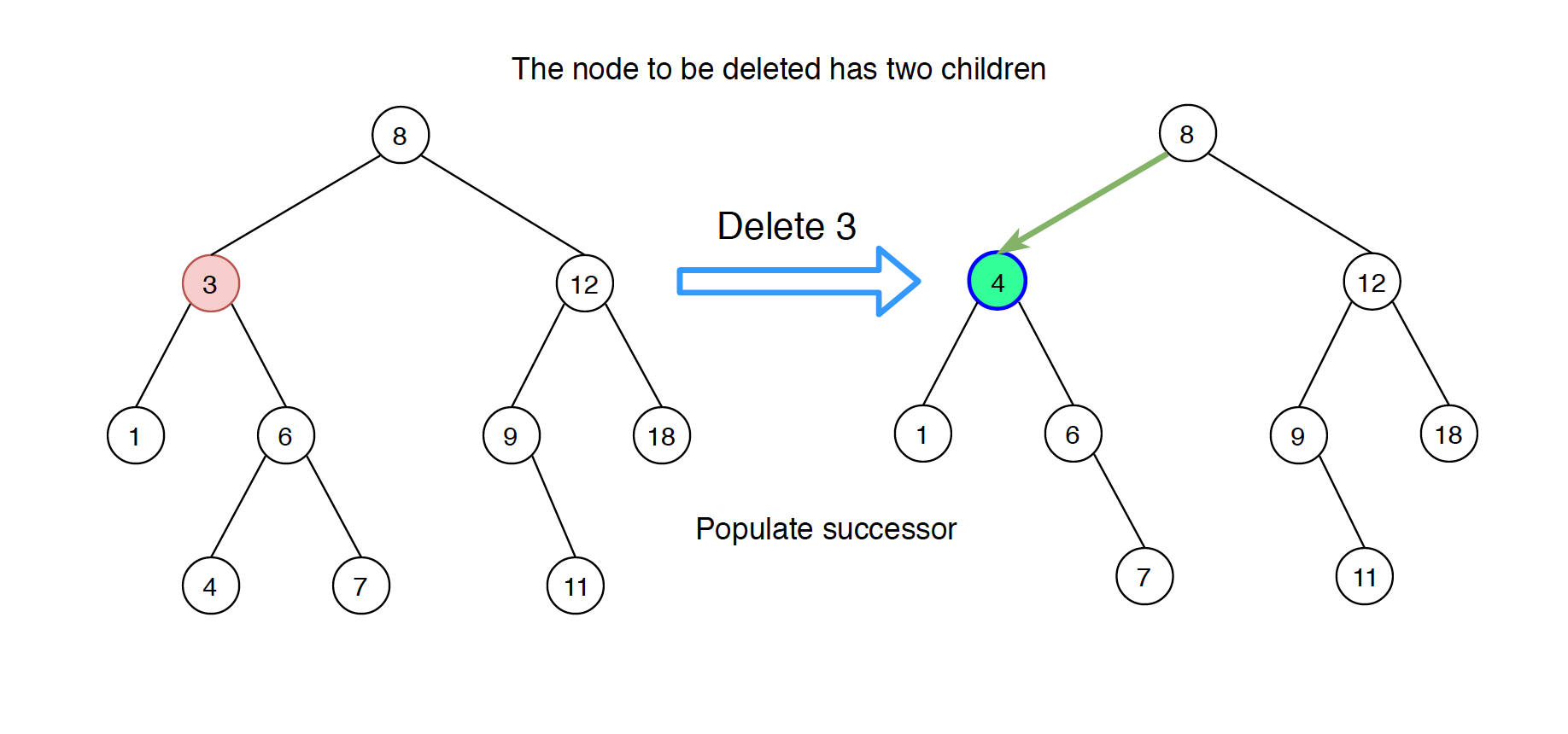Find inorder successor of the node. Replace the node with its successor and delete the successor from its original parent.
2) Populate predecessor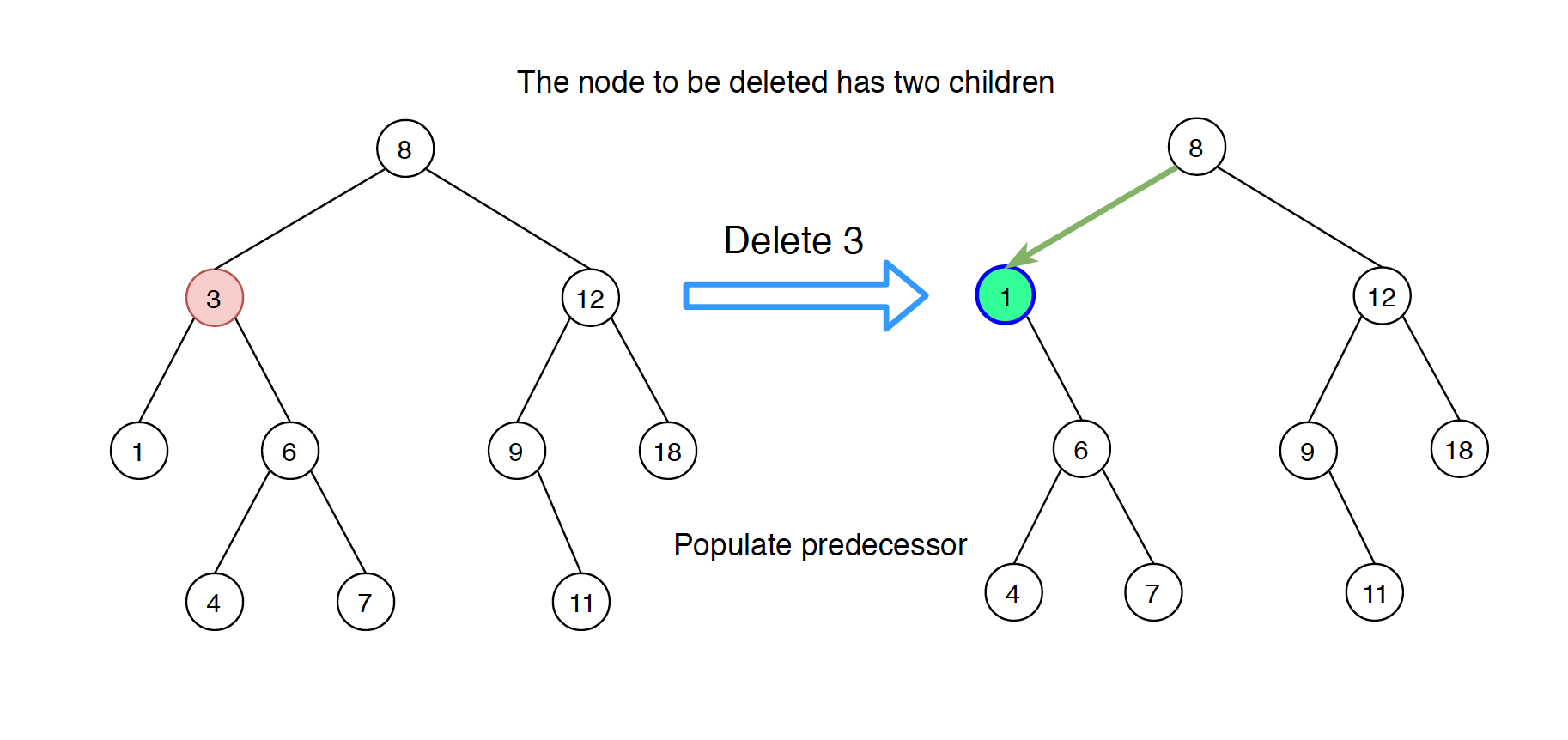Find inorder predecessor of the node. Replace the node with its predecessor and delete the predecessor from its original parent.

The following implementation populates the successor of the deleted node.

public boolean delete(int val) {
BSTNode parent = root;
BSTNode current = root;
boolean isLeftChild = false;

while (current.val != val){
parent = current;
if (current.val > val){
isLeftChild = true;
current = current.left;
} else {
isLeftChild = false;
current = current.right;
}
if (current == null) {
return false;
}
}

//if i am here that means we have found the node
//Case 1: if node to be deleted has no children(leaf)
if (current.left == null && current.right == null) {
if (current == root) {
root = null;
}
if (isLeftChild == true) {
parent.left = null;
} else {
parent.right = null;
}
}
//Case 2 : if node to be deleted has only one child
else if (current.right == null) {
if (current == root) {
root = current.left;
} else if(isLeftChild) {
parent.left = current.left;
} else {
parent.right = current.left;
}
}
else if (current.left == null) {
if (current == root) {
root = current.right;
} else if (isLeftChild) {
parent.left = current.right;
}else{
parent.right = current.right;
}
}
//Case 3 : if node to be deleted has two children
else if (current.left != null && current.right != null){
//now we have found the minimum element in the right sub tree
BSTNode successor = getSuccessor(current);
if (current == root) {
root = successor;
} else if (isLeftChild) {
parent.left = successor;
}else{
parent.right = successor;
}
successor.left = current.left;
}
return true;
}

private BSTNode getSuccessor(BSTNode deleleNode) {
BSTNode successsor =null;
BSTNode successsorParent =null;
BSTNode current = deleleNode.right;
while (current != null){
successsorParent = successsor;
successsor = current;
current = current.left;
}
//check if successor has the right child, it cannot have left child for sure
// if it does have the right child, add it to the left of successorParent.
//      successsorParent
if (successsor != deleleNode.right) {
successsorParent.left = successsor.right;
successsor.right = deleleNode.right;
}
return successsor;
}


## 5. Balanced Binary Search Tree

In some cases, we need to convert a normal BST tree to a balanced BST.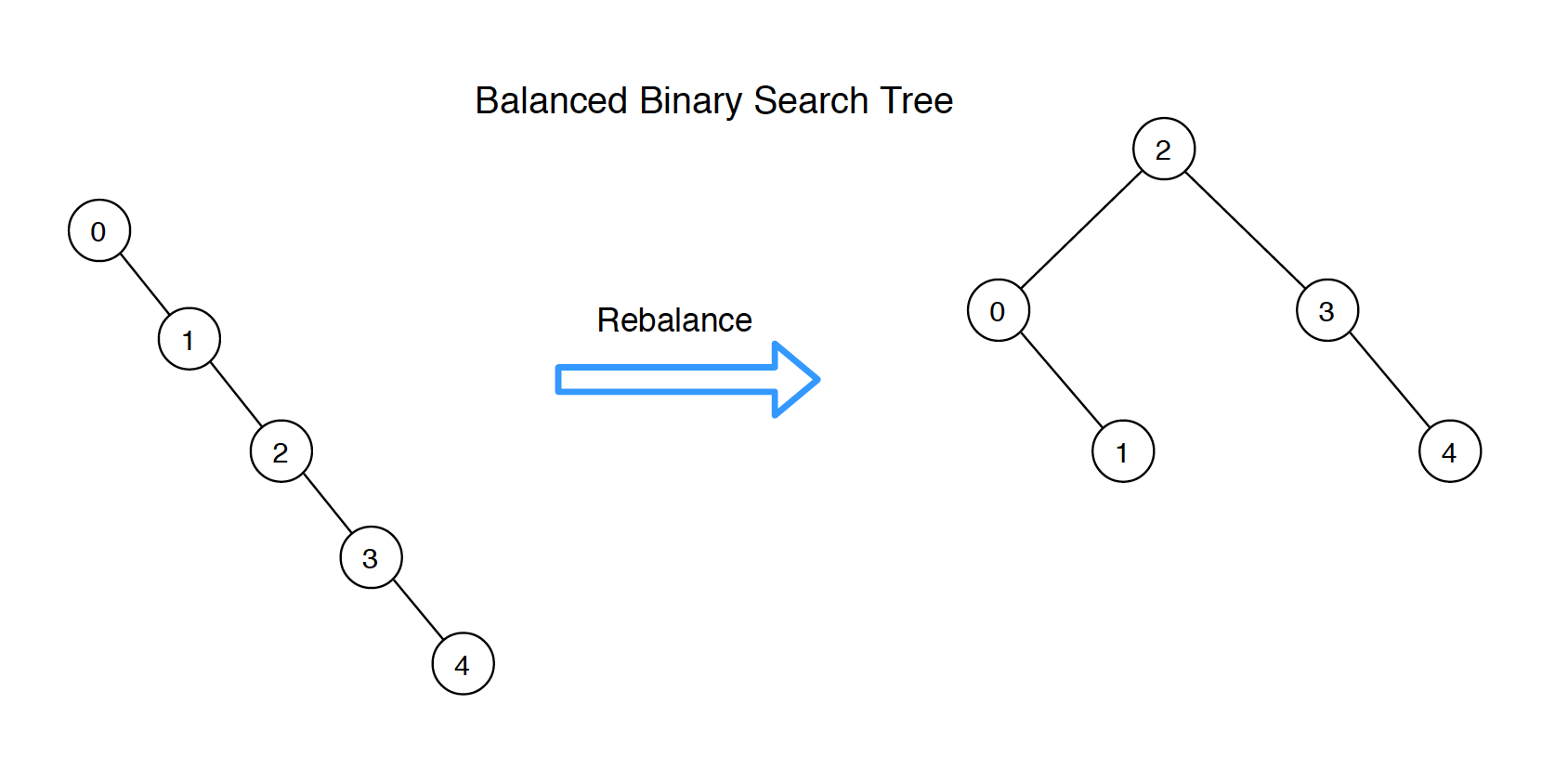We can convert it with the following two steps.

• Get the sorted node list from existing bst tree by traversing it inorder.
• Binary construct the balanced BST with the sorted node list.
public BSTNode rebalanceBST(BSTNode root) {
List<BSTNode> list = inorder(root);
if (list == null || list.size() == 0) {
return null;
}

return sortedListToBST(list, 0, list.size() - 1);
}

// build bst with give node list.
private BSTNode sortedListToBST(List<BSTNode> list, int start, int end) {
if (start > end) {
return null;
}

int mid = start + (end - start) / 2;
BSTNode root = list.get(mid);

root.left = sortedListToBST(list, start, mid - 1);
root.right = sortedListToBST(list, mid + 1, end);
return root;
}

// get the sorted node list of bst tree
public List<BSTNode> inorder(BSTNode root) {
List<BSTNode> res = new ArrayList<BSTNode>();

if (root == null) {
return res;
}

List<BSTNode> left = inorder(root.left);
List<BSTNode> right = inorder(root.right);

return res;
}


## 6. Binary Search Tree Iterator

public class BSTIterator {
private Stack<BSTNode> stack = new Stack<BSTNode>();
private BSTNode curr;
public BSTIterator(BSTNode root) {
curr = root;
}

/** @return whether we have a next smallest number */
public boolean hasNext() {
return (curr != null || !stack.isEmpty());
}

/** @return the next smallest number */
public int next() {
while (curr != null) {
stack.push(curr);
curr = curr.left;
}

curr = stack.pop();
int res = curr.val;
curr = curr.right;
return res;
}
}